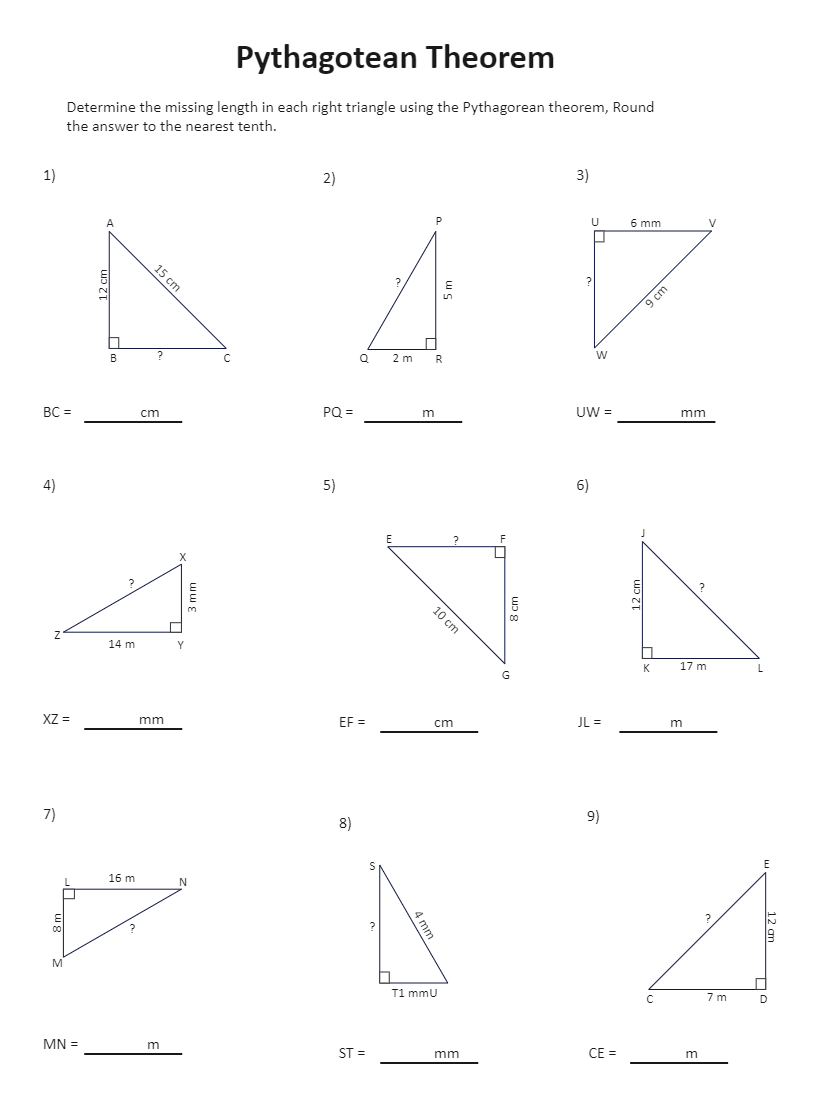Template Community / Pythagorean Theorem Worksheet

# Pythagorean Theorem Worksheet

Use Template9
0
Report
Publish time：02-09-2022
Tag:
science diagram
Mathematics Diagram
The Pythagoras Theorem states that if a triangle is right-angled, then the square of the hypotenuse is equal to the sum of the squares of the other two sides. Following is the Pythagorean Theorem Assignment, where the students have to determine the missing length in each right triangle using the Pythagorean theorem. Once they determine the missing value, they must enter it in the blank area. Use EdrawMax to create such mathematics worksheets. The 2D worksheet maker lets you import your designs that you can later share with the students or their family members using the built-in sharing feature.
CreatorKiraaaaPost
Recommended Templates